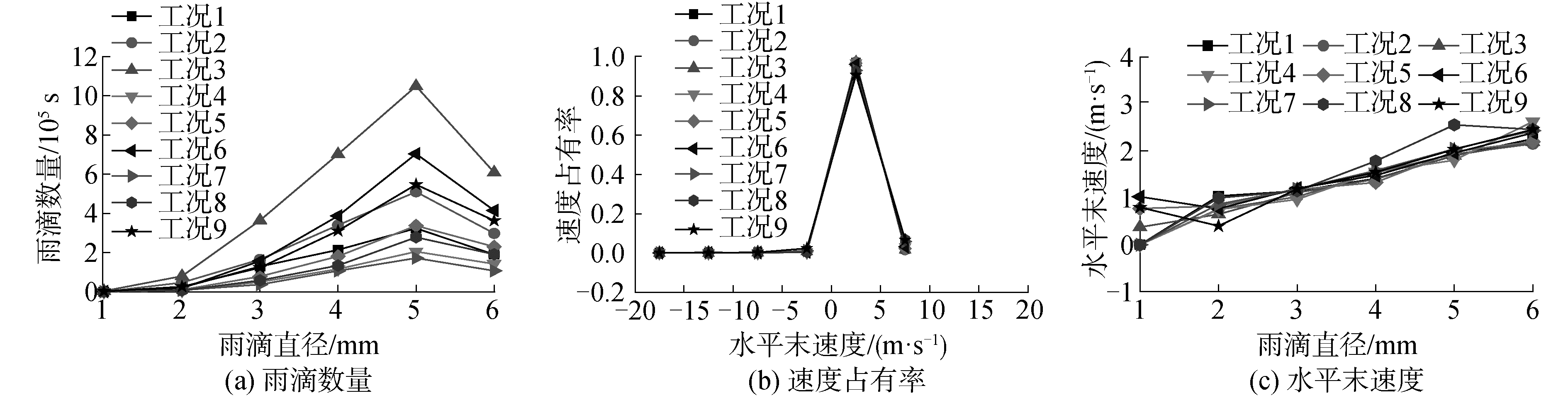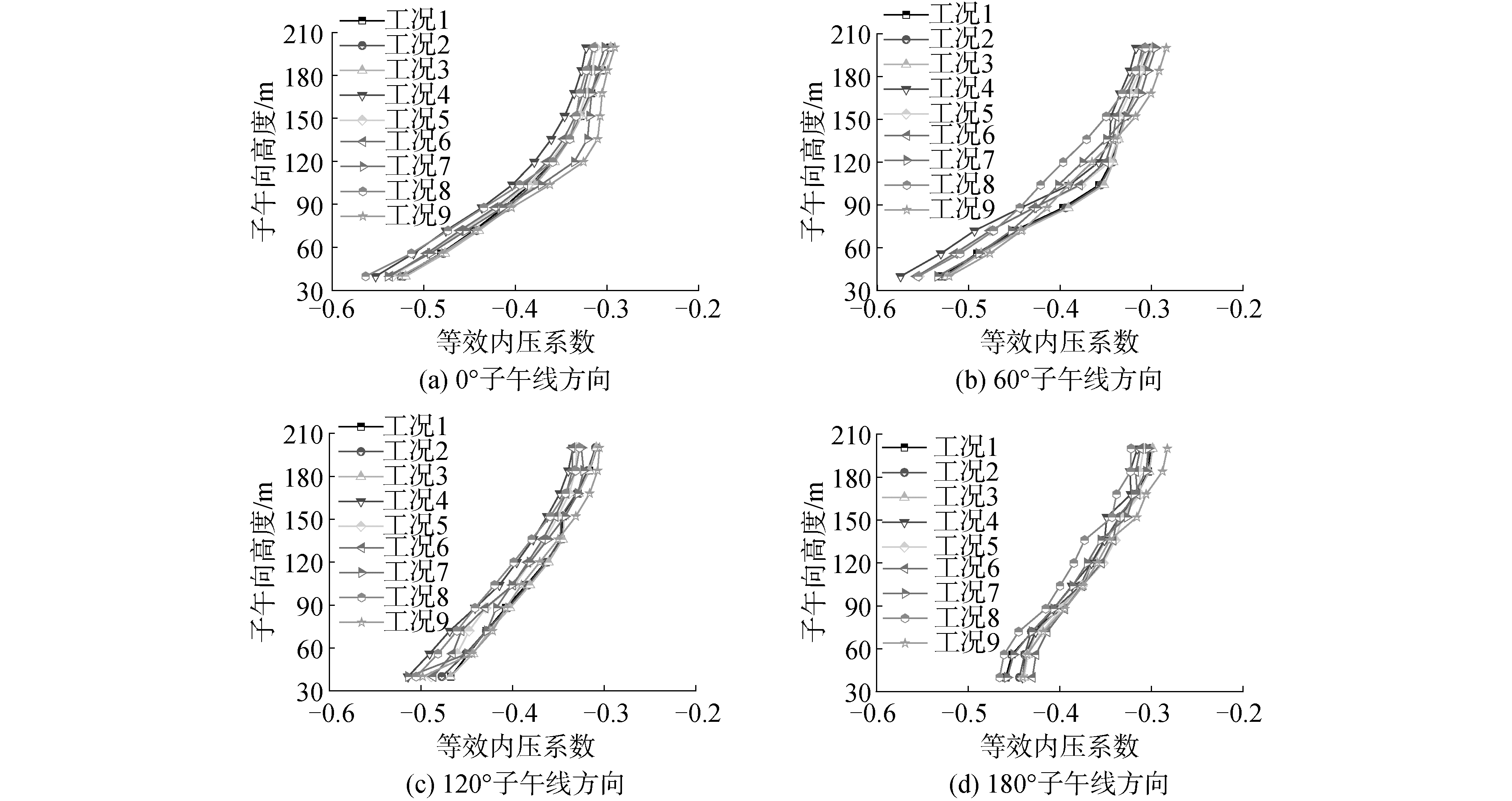﻿ 风-雨荷载下大型冷却塔内压作用
«上一篇文章快速检索 高级检索

 哈尔滨工程大学学报2019, Vol. 40Issue (5): 926-931  DOI: 10.11990/jheu.2017120540

### 引用本文YU Wenlin, KE Shitang. Internal pressure effect of large cooling tower under wind-rain loads[J]. Journal of Harbin Engineering University, 2019, 40(5), 926-931. DOI: 10.11990/jheu.201712054.### 文章历史

1. 南京航空航天大学 土木工程系, 江苏 南京 210016;
2. 中国能源建设集团 江苏省电力设计院有限公司, 江苏 南京 211102

Internal pressure effect of large cooling tower under wind-rain loads
YU Wenlin 1,2, KE Shitang 11. Department of Civil Engineering, Nanjing University of Aeronautics and Astronautics, Nanjing 210016, China;
2. China Energy Engineering Group Jiangsu Power Design Institute Co., Ltd., Nanjing 211102, China
Abstract: In this study, the two-phase flow of wind and rain was simulated and the aerodynamic force on internal surface was studied to investigate the effect of rainfall on large cooling towers in stormy weather. First, considering a 210 m high super large cooling tower as an example, and the wind-rain coupling algorithm as the core, the wind and rain fields were simulated using continuous and discrete phase models based on CFD (computational fluid dynamics), respectively. Furthermore, the influence laws of nine different combinations of wind speed-rain intensity on the rainfall, rain load, and equivalent internal pressure coefficient on the internal surface of tower were studied, thereby revealing the action mechanisms of wind speed streamline and rain drops moving in a trajectory inside the tower. Finally, the most unfavorable conditions and the internal pressure coefficient distribution characteristics were extracted. Results show that the rain-induced pressure coefficient of the leeward zone on top of the internal surface under wind-rain loads cannot be ignored, and that the maximum value can reach 0.003 8. The main conclusions can provide reference for determining the inner surface pressure of such cooling tower under extreme weather conditions.
Keywords: cooling tower    computational fluid dynamics    wind-rain coupling algorithm    two-phase flow simulation    parameter analysis    surface load    internal pressure effect    aerodynamic force distribution

1 风-雨耦合算法 1.1 降雨强度等级化分表 1 降雨强度等级划分 Table 1 Grades of rainfall intensity

 $n\left( {{D_{\rm{p}}}} \right) = {N_0}{{\rm{e}}^{ - \lambda {D_{\rm{p}}}}}$ (1)

 $\lambda = 4.1 \times {R^{ - 0.21}}$ (2)

 $F(\tau ) = \frac{1}{{6\tau }}{\rho _{\rm{p}}}\pi D_{\rm{p}}^{\rm{3}}{v_{\rm{s}}} = \frac{{2{v_{\rm{s}}}}}{{6{D_{\rm{p}}}}}{\rho _{\rm{p}}}\pi D_{\rm{p}}^{\rm{3}}{v_{\rm{s}}} = \frac{1}{3}{\rho _{\rm{p}}}\pi D_{\rm{p}}^{\rm{2}}v_{\rm{s}}^{\rm{2}}$ (3)

1.2 工程简介与工况设置表 2 工况组合划分表 Table 2 Division of working condition combination
1.3 风-雨耦合数值模拟Download: 图 1 核心区网格划示意 Fig. 1 Grid division on core area

 $\frac{{{\rm{d}}{u_{\rm{p}}}}}{{{\rm{d}}t}} = {F_{\rm{D}}}\left( {u - {u_{\rm{p}}}} \right) + \frac{{g\left( {{\rho _{\rm{p}}} - \rho } \right)}}{{{\rho _{\rm{p}}}}} + F$ (4)

 ${F_{\rm{D}}} = \frac{{18\mu }}{{{\rho _{\rm{p}}}D_{\rm{p}}^{\rm{2}}}}\frac{{{C_{\rm{D}}}Re}}{{24}}$ (5)

 $Re = \frac{{\rho {D_{\rm{p}}}\left| {{u_{\rm{p}}} - u} \right|}}{\mu }$ (6)

 $\frac{{\partial \rho }}{{\partial t}} + \nabla (\rho u) = {S_{\rm{m}}}$ (7)
 $\frac{\partial}{\partial t}(\rho u)+\nabla(\rho u u)=-\nabla_{p}+\nabla\left(\tau_{s}\right)+\rho g+F$ (8)

 ${\tau _{\rm{s}}} = \mu \left[ {\left( {\nabla u + \nabla {u_{\rm{v}}}} \right) - \frac{2}{3}} \right]\nabla u\mathit{\boldsymbol{I}}$ (9)

2 风-雨场模拟结果对比分析 2.1 风场分析Download: 图 2 冷却塔三维风速流线图 Fig. 2 Three-dimensional wind speed streamline of cooling towers
2.2 雨场分析Download: 图 3 风雨场中雨滴运动轨迹示意图 Fig. 3 Raindrop motion trajectory in rain and wind field

1) 受来流风水平方向力影响，所有雨滴均斜向降落，一部分雨滴撞击在塔筒迎风面，其余掠过冷却塔沿气流在塔筒两侧分离，切仅有少量雨滴附着在两侧壁，大多数随来流风运动至尾部区域；

2) 冷却塔高度范围上部前端流场中的雨滴在风力、重力和空气阻力共同作用下，穿过冷却塔出风口进入冷却塔内部，并以一定速度撞击至中上部背风区域，且降雨强度愈大，内壁面雨滴收集愈多；

3) 随着风速的增大，略过冷却塔顶部向塔体后方行进的雨滴逐渐增多，使得进入冷却塔内部的雨滴数量急剧减少，此外，高风速下出风口气流向上作用力相比低风速下较大，此现象将更为显著。Download: 图 4 雨滴数量速度、占有率与水平末速度分布曲线 Fig. 4 Distribution curves of raindrop number, speed share and velocityDownload: 图 5 各工况冷却塔内表面雨滴和雨致内压系数三维分布示意 Fig. 5 Three-dimensional distribution of raindrop and rain-induced internal pressure coefficient on internal surface of cooling tower
2.3 等效内压系数分析

 ${C_{{\rm{e}}i}} = {C_{{\rm{w}}i}} + {C_{{\rm{r}}i}}$ (10)Download: 图 6 冷却塔典型断面环向等效内压系数对比曲线 Fig. 6 Comparison curves of equivalent internal pressure coefficient in typical sections of cooling towerDownload: 图 7 冷却塔典型子午线等效内压系数对比曲线 Fig. 7 Comparison curves of equivalent internal pressure coefficient in typical meridians of cooling tower
3 结论

1) 受来流风水平力影响，所有雨滴均斜向降落，部分雨滴以一定速度撞击至塔筒内表面中上部背风区域，大多数随来流风运动至尾部区域。

2) 附着在结构内表面的雨滴数量随着风速的逐渐增大而减少，随着雨强的逐渐增大而增加，附着数量最多的为风速20 m/s、雨强200 mm/h工况。

3) 雨滴主要附着在塔筒内表面顶部背风区域，该区域雨荷载对内表面的压力影响不可小视。

4) 由于附加雨荷载效应，塔筒背风区域等效内压系数出现减小现象，同时不同子午线方向等效内压系数均随高度的增大逐渐减小。

  余玮, 柯世堂. 考虑风热耦合作用特大型冷却塔内吸力及流场作用机理研究[J]. 工程力学, 2017, 34(12): 112-119, 142. YU Wei, KE Shitang. Research on the internal suction and flow field mechanism for super-large cooling towers under wind-thermal coupling[J]. Engineering mechanics, 2017, 34(12): 112-119, 142. (0)  电力行业电力规划设计标准化技术委员会. DLT 5339-2006, 火力发电厂水工设计规范[S].北京: 中国电力出版社, 2006. Technical Committee for Standardization of Electric Power Planning and Design in Electric Power Industry. DLT 5339-2006, Code for hydraulic design of fossil fuel power plants[S]. Beijing: China Electric Power Press, 2006. (0)  VGB PowerTech. VGB-R610Ue, VGB-Guideline: structural design of cooling towers[S]. Essen: BTR Bautechnik Bei Kuhlturmen, 2005. (0)  余文林, 柯世堂. 基于风-雨双向耦合的大型冷却塔结构响应[J]. 哈尔滨工业大学学报, 2018, 50(12): 114-118. YU Wenlin, KE Shitang. Structural responses of large cooling tower based on bidirectional coupling between wind and rain[J]. Journal of Harbin Institute of Technology, 2018, 50(12): 114-118. DOI:10.11918/j.issn.0367-6234.201804026 (0)  柯世堂, 杜凌云, 侯宪安. 考虑百叶窗透风率超大型冷却塔内吸力风振系数研究[J]. 建筑结构学报, 2018, 39(8): 36-44. KE Shitang, DU Lingyun, HOU Xian'an. Research on influence of louver ventilation rates on internal wind vibration coefficient for super large cooling towers[J]. Journal of building structures, 2018, 39(8): 36-44. (0)  邹云峰, 何旭辉, 陈政清, 等. 超大型冷却塔内表面风荷载风洞试验与数值模拟研究[J]. 空气动力学学报, 2015, 33(5): 697-705. ZOU Yunfeng, HE Xuhui, CHEN Zhengqing, et al. Wind tunnel test and numerical simulation study on internal wind loading for super large cooling tower[J]. Acta aerodynamica sinica, 2015, 33(5): 697-705. (0)  董国朝, 张建仁, 蔡春声, 等. 考虑内部构件影响的超大型冷却塔内压系数研究[J]. 工程力学, 2016, 33(4): 77-83. DONG Guochao, ZHANG Jianren, CAI Chunsheng, et al. Study on internal surface pressure coefficient of super-large cooling tower with different internal main components[J]. Engineering mechanics, 2016, 33(4): 77-83. (0)  BLOCKEN B, CARMELIET J. Spatial and temporal distribution of driving rain on a low-rise building[J]. Wind and structures, 2002, 5(5): 441-462. DOI:10.12989/was.2002.5.5.441 (0)  XIN Dabo, LI Hui, WANG Liang, et al. Experimental study on static characteristics of the bridge deck section under simultaneous actions of wind and rain[J]. Journal of wind engineering and industrial aerodynamics, 2012, 107-108: 17-27. DOI:10.1016/j.jweia.2012.03.002 (0)  FU Xing, LI Hongnan, LI Gang. Fragility analysis and estimation of collapse status for transmission tower subjected to wind and rain loads[J]. Structural safety, 2016, 58: 1-10. DOI:10.1016/j.strusafe.2015.08.002 (0)  WANG L Y, XU Y L. Active stiffness control of wind-rain-induced vibration of prototype stay cable[J]. International journal for numerical methods in engineering, 2008, 74(1): 80-100. DOI:10.1002/(ISSN)1097-0207 (0)  MCFARQUHAR G M, LIST R. The raindrop mean free path and collision rate dependence on rainrate for Three-Peak equilibrium and Marshall-Palmer distributions[J]. Journal of the atmospheric sciences, 1991, 48(17): 1999-2003. DOI:10.1175/1520-0469(1991)048<1999:TRMFPA>2.0.CO;2 (0)  BI J H, QIAO H Y, NIKITAS N, et al. Numerical modelling for rain wind induced vibration of cables with longitudinal ribs[J]. Journal of wind engineering & industrial aerodynamics, 2018, 178: 69-79. (0)  KE Shitang, YU Wenlin, GE Yaojun. Wind load characteristics and action mechanism on internal and external surfaces of super-large cooling towers under wind-rain combined effects[J]. Mathematical problems in engineering, 2018, 2018: 2921709. (0)